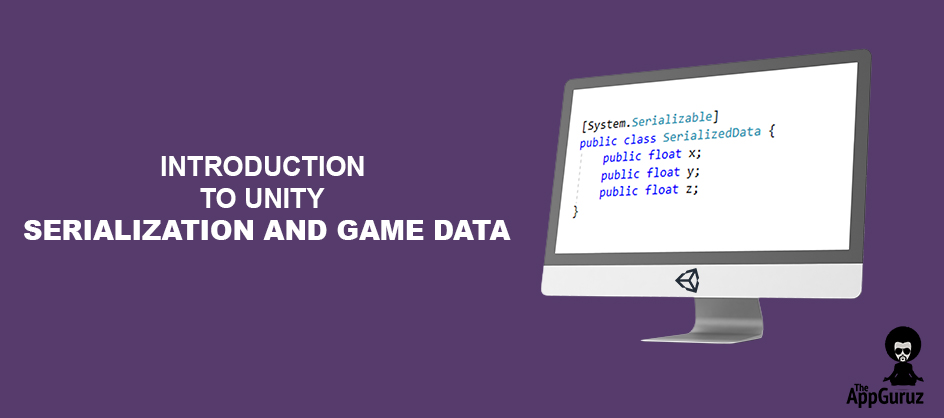## Objective

The main Objective of this blog post to learn about Unity Serialization and Game Data.

What is Serialization?

What is Serialized data?

How to Serialize your own data?

How Unity dose Serialization?

Do you have the same questions in your mind?

Then you are at the right place.

Let’s get one ride to it.

According to Unity API Documentation,

• "Serialization is the process of converting the state of an object to a set of bytes in order to store  the object into memory, a database or a file."

Here, the pictorial view of the Serialization process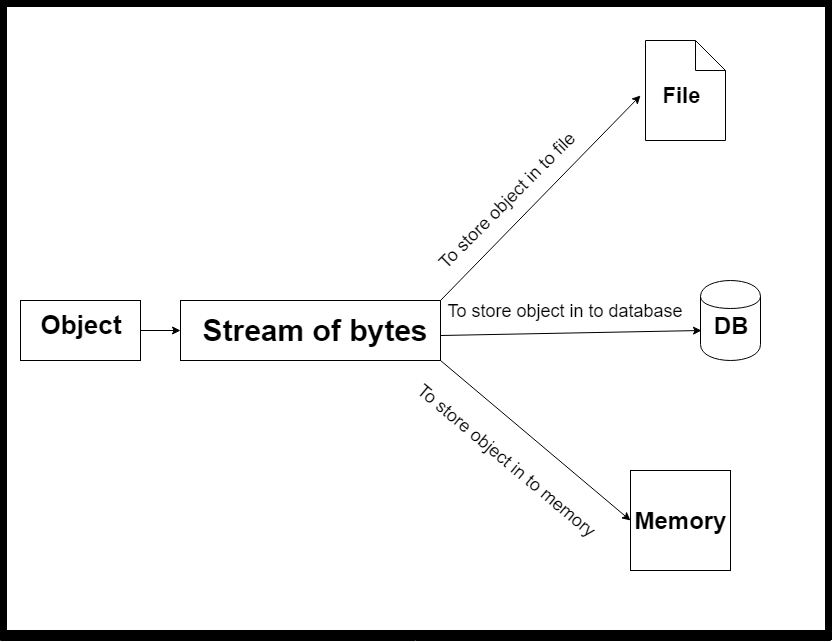The process of storing the state of the object as “Serialization”

and

The reverse process of building an object out of a file "Deserialization".

A pictorial view of the Deserialization process.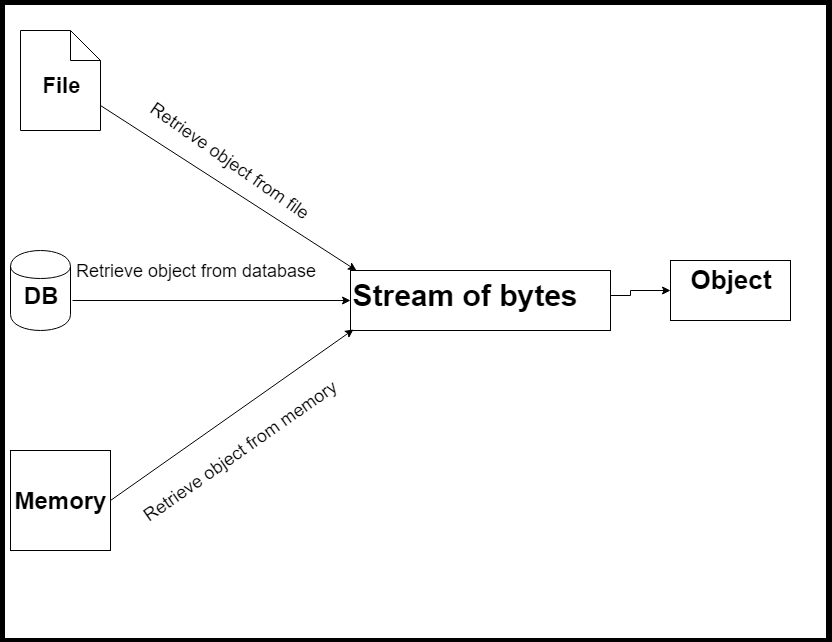The data that Unity derives when it’s trying to make copies or save copies of things that derive from UnityEngine. Object, Scene, in Prefabs.

These are most commonly saved on disk but used in memory.

Let’s see which types of data can be Serialized

• Be public, or have [SerializeField] attribute
• public float pi = 3.14f;
• SerializeField] private int a = 3;
• Not be static
• public static int myStatic = 13;(will not get serialized...)
• Not be const
• public const int myConst=15;(will not get serialized...)

Let’s take one look on which field types that we can serialize

• Custom non abstract classes with [Serializable] attribute
``````[System.Serializable]
public class SerializedDataCity  {
public string name;
}
``````
• Custom structs with [Serializable] attribute.
• References to objects that derive from UnityEngine.Object
• public SerializedData serializedData= new SerializedData ();
• Primitive data types
• int,float,double,bool,string,etc
• Array of a field type we can serialize
• List<T> of a field type we can serialize
``public List<SerializedDataCity> cities;``

Let’s take an example,

Step 1)

• Create an Empty gameObject name it as you like.

Step 2)

• Create a C# script name it as you like.

Write the following code in to you script.

``````public class SerializedDemo : MonoBehaviour {
[SerializeField] private int a = 3;
[SerializeField] private int b = 4;
public float pi = 3.14f;
private int myPrivate = 12;
public static int myStatic = 13;
public const int myConst=15;
void Start () {
}
void Update () {
if (Input.GetMouseButtonDown (0)) {
a = 10;
b = 14;
pi = 9;
myPrivate = 45;
myStatic = 89;
Debug.Log("Object Instantiate....");
GameObject.Instantiate(gameObject);
}
}
}
``````

Step 3)

• Assign it to Empty gameObject.

In above code only variables,

• [SerializeField] private int a = 3;
• [SerializeField] private int b = 4;
• public float pi = 3.14f;

Get serialized because, they are the only fields according to the rules for Serialization field.

Now let’s see in Unity,

One way to show this is by taking a look at the inspector window of this script.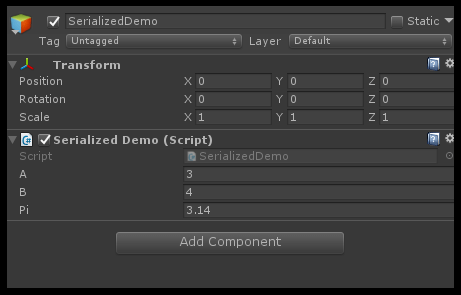Which  shows only serialized fields. This is one of the way to see the serialized field.

Let’s take another way,

To see the serialization is by cloning the object.

The Instantiate() method uses the Unity serialization process.

Wait…

How the this method uses the Unity serialization process?

When you instantiate a copies of a gameObject, Unity Serializes the original copy.

Recreate the gameObject and component structure of original gameObject and then feeds the serialized data.

Confused? :-S

Let’s try to understand it by our example.

In our example,

When the mouse  left button is clicked, method should modify the current object and create another object in the scene.

Below code will do this for us in our example.

``````if (Input.GetMouseButtonDown (0)) {
a = 10;
b = 14;
pi = 9;
myPrivate = 45;
myStatic = 89;
Debug.Log("Object Instantiate....");
GameObject.Instantiate(gameObject);
}

``````

Let’s see what happens when cloning object,

• Unity Serialized the original copy.
• Unity Serialization process recreates the gameObject and component structure of original gameObject.
• Latter in this process it will feed the serialized data.

In our example,

We know variables "a","b", and "pi" are serialized field,When object clone these three field feed by serialized data.

Take a look of console,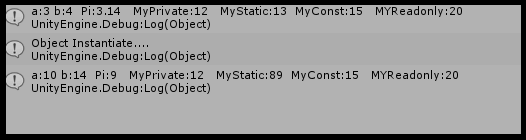Here, we can see that values of variables "a", "b" and "pi" get serialized and clone object get that serialized data.

Other variables "myPrivate", "myConst" and "myReadonly" have the same value as original object because they’re not serialized fields.

But wait,

In the clone object the value of “myStatic” variable also change.

Does that mean static fields are serializable?

Even though its behavior leads us to conclude that, static fields belong to the class, and not to an instance of it. ;)

Uptill now you may got idea about the classes that inherit from Unity.object or primitive data type that is serializable type.

What if I want to serialize a custom class which is not inherit from Unity.object?

Here is the simple solution, add the [System.Serializable] modifier to the class

Step 1)

• Create an Empty gameObject name it as you like.

Step 2)

• Create C# script name it as you like.

Write the following code into it. (MyDatabase.cs)

``````public class MyDatabase : MonoBehaviour {
public List<SerializedDataCity> cities;
}
``````

Step 3)

• Assign it Empty gameObject.

Step 4)

• Create C# script name it as you like.

Write following code in your script. (SerializesDataCity.cs).

``````[System.Serializable]
public class SerializedDataCity  {
public string name;
}
``````

Here, you can see the list of city data shown in  Inspector window of empty gameObject created in step 1.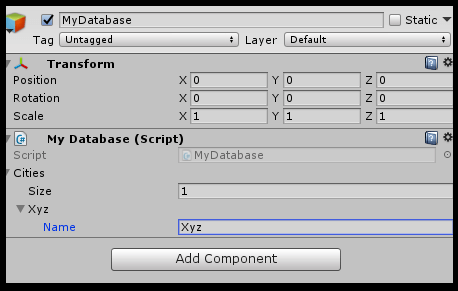I hope now you got the basic idea about serialization.

For more understanding,

Let’s take one realtime demo, I'm sure it will clear all your doubts. :)

In example, player position (x,y,z component) store for next play.

Step 1)

• Create 3D gameObject name it player.

Step 2)

• Crate C# script name it PlayerMotion.

Write following code into PlayerMotion.cs.

``````public class PlayerMotion : MonoBehaviour {
public float speed=0.1f;
Vector3 position;
void Update () {
if (Input.GetKey (KeyCode.RightArrow)) {
position = transform.position;
position.x += speed;
transform.position = position;
}
if (Input.GetKey (KeyCode.LeftArrow)) {
position = transform.position;
position.x -= speed;
transform.position = position;
}
if (Input.GetKey (KeyCode.UpArrow)) {
position = transform.position;
position.y += speed;
transform.position = position;
}
if (Input.GetKey (KeyCode.DownArrow)) {
position = transform.position;
position.y -= speed;
transform.position = position;
}
}
}
``````

Step 3)

• Assign it to Player.

Step 4)

• Create an Empty gameObject name it as you like.

Step 5)

• Create C# script name it as like.

Write following code into  your script (SavePlayerPosition.cs)

``````public class SavePlayerPosition : MonoBehaviour {

public GameObject player;
public SerializedData serializedData ;

string savePositionData;
string restorePosition;
void Start () {
serializedData= new SerializedData ();
RestorePosition ();

}

void Update () {
if (Input.GetKeyDown (KeyCode.S)) {
SavePosition ();
}
}
void SavePosition(){
serializedData.x = player.transform.position.x;
serializedData.y = player.transform.position.y;
serializedData.z = player.transform.position.z;
savePositionData = JsonUtility.ToJson (serializedData);
PlayerPrefs.SetString ("PlayerPosition",savePositionData);
Debug.Log (savePositionData);
}

void RestorePosition(){
restorePosition = PlayerPrefs.GetString ("PlayerPosition");
SerializedData	serializedData1 = JsonUtility.FromJson<SerializedData> (restorePosition);
if (serializedData1 != null) {
Vector3 position = new Vector3 ();
position.x = serializedData1.x;
position.y = serializedData1.y;
position.z = serializedData1.z;
player.transform.position = position;
}
}

}``````

Step 6)

• Assign it to Empty gameObject.

This script saves player’s position by pressing ‘S’ key from keyboard and move around scene using arrow keys.

Step 7)

• Create C# script name it as you like.

Write following code into your script.

``````[System.Serializable]
public class SerializedData {
public float x;
public float y;
public float z;
}
``````

This is serialized class which store player position.

Finally, let’s summarize the modifiers involved in serialization.

• Use [System.Serializable] on a class or struct definition if you want it to be serialized;
• Publics fields are serialized by default, but only if its type is serializable (constants, static and readonly fields are not serialized);
• Use [SerializeField] if you wish to serialize a private field;
• Use [NonSerialized] if you want to avoid serialization on a public field;
• Use [HideInInspector] if you want to serialize but not expose the field in the inspector;

If you need to ask something or share any ideas about this topic just drop a comment in the comment section. We’ll be there with you. :)